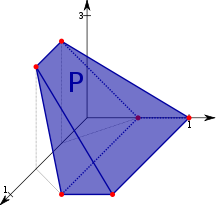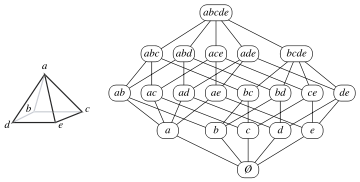# Convex polytopeA 3-dimensional convex polytope

A convex polytope is a special case of a polytope, having the additional property that it is also a convex set of points in the n-dimensional space Rn. Some authors use the terms "convex polytope" and "convex polyhedron" interchangeably, while others prefer to draw a distinction between the notions of a polyhedron and a polytope.

In addition, some texts require a polytope to be a bounded set, while others (including this article) allow polytopes to be unbounded. The terms "bounded/unbounded convex polytope" will be used below whenever the boundedness is critical to the discussed issue. Yet other texts treat a convex n-polytope as a surface or (n-1)-manifold.

Convex polytopes play an important role both in various branches of mathematics and in applied areas, most notably in linear programming.

A comprehensive and influential book in the subject, called Convex Polytopes, was published in 1967 by Branko Grünbaum. In 2003 the 2nd edition of the book was published, with significant additional material contributed by new writers.

In Grünbaum's book, and in some other texts in discrete geometry, convex polytopes are often simply called "polytopes". Grünbaum points out that this is solely to avoid the endless repetition of the word "convex", and that the discussion should throughout be understood as applying only to the convex variety.

A polytope is called full-dimensional if it is an n-dimensional object in Rn.

## ExamplesWikimedia Commons has media related to Polyhedra.
• Many examples of bounded convex polytopes can be found in the article "polyhedron".
• In the 2-dimensional case the full-dimensional examples are a half-plane, a strip between two parallel lines, an angle shape (the intersection of two non-parallel half-planes), a shape defined by a convex polygonal chain with two rays attached to its ends, and a convex polygon.
• Special cases of an unbounded convex polytope are a slab between two parallel hyperplanes, a wedge defined by two non-parallel half-spaces, a polyhedral cylinder (infinite prism), and a polyhedral cone (infinite cone) defined by three or more half-spaces passing through a common point.

## Definitions

A convex polytope may be defined in a number of ways, depending on what is more suitable for the problem at hand. Grünbaum's definition is in terms of a convex set of points in space. Other important definitions are: as the intersection of half-spaces (half-space representation) and as the convex hull of a set of points (vertex representation).

### Vertex representation (Convex hull)

In his book Convex polytopes, Grünbaum defines a convex polytope as a compact convex set with a finite number of extreme points:

A set K of Rn is convex if, for each pair of distinct points a, b in K, the closed segment with endpoints a and b is contained within K.

This is equivalent to defining a bounded convex polytope as the convex hull of a finite set of points, where the finite set must contain the set of extreme points of the polytope. Such a definition is called a vertex representation (V-representation or V-description). For a compact convex polytope, the minimal V-description is unique and it is given by the set of the vertices of the polytope.

### Intersection of half-spaces

A convex polytope may be defined as an intersection of a finite number of half-spaces. Such definition is called a half-space representation (H-representation or H-description). There exist infinitely many H-descriptions of a convex polytope. However, for a full-dimensional convex polytope, the minimal H-description is in fact unique and is given by the set of the facet-defining halfspaces.

A closed half-space can be written as a linear inequality:where n is the dimension of the space containing the polytope under consideration. Hence, a closed convex polytope may be regarded as the set of solutions to the system of linear inequalities:where m is the number of half-spaces defining the polytope. This can be concisely written as the matrix inequality:where A is an m×n matrix, x is an n×1 column vector of variables, and b is an m×1 column vector of constants.

An open convex polytope is defined in the same way, with strict inequalities used in the formulas instead of the non-strict ones.

The coefficients of each row of A and b correspond with the coefficients of the linear inequality defining the respective half-space. Hence, each row in the matrix corresponds with a supporting hyperplane of the polytope, a hyperplane bounding a half-space that contains the polytope. If a supporting hyperplane also intersects the polytope, it is called a bounding hyperplane (since it is a supporting hyperplane, it can only intersect the polytope at the polytope's boundary).

The foregoing definition assumes that the polytope is full-dimensional. If it is not, then the solution of Axb lies in a proper affine subspace of Rn and discussion of the polytope may be constrained to this subspace.

In general the intersection of arbitrary half-spaces need not be bounded. However if one wishes to have a definition equivalent to that as a convex hull, then bounding must be explicitly required.

#### Finite basis theorem

The finite basis theorem is an extension of the notion of V-description to include infinite polytopes. The theorem states that a convex polyhedron is the convex sum of its vertices plus the conical sum of the direction vectors of its infinite edges.

## Properties

Every (bounded) convex polytope is the image of a simplex, as every point is a convex combination of the (finitely many) vertices. However, polytopes are not in general isomorphic to simplices. This is in contrast to the case of vector spaces and linear combinations, every finite-dimensional vector space being not only an image of, but in fact isomorphic to, Euclidean space of some dimension (or analog over other fields).

### The face lattice

A face of a convex polytope is any intersection of the polytope with a halfspace such that none of the interior points of the polytope lie on the boundary of the halfspace. If a polytope is d-dimensional, its facets are its (d  1)-dimensional faces, its vertices are its 0-dimensional faces, its edges are its 1-dimensional faces, and its ridges are its (d  2)-dimensional faces.

Given a convex polytope P defined by the matrix inequality, if each row in A corresponds with a bounding hyperplane and is linearly independent of the other rows, then each facet of P corresponds with exactly one row of A, and vice versa. Each point on a given facet will satisfy the linear equality of the corresponding row in the matrix. (It may or may not also satisfy equality in other rows). Similarly, each point on a ridge will satisfy equality in two of the rows of A.The face lattice of a square pyramid, drawn as a Hasse diagram; each face in the lattice is labeled by its vertex set.

In general, an (n  j)-dimensional face satisfies equality in j specific rows of A. These rows form a basis of the face. Geometrically speaking, this means that the face is the set of points on the polytope that lie in the intersection of j of the polytope's bounding hyperplanes.

The faces of a convex polytope thus form an Eulerian lattice called its face lattice, where the partial ordering is by set containment of faces. The definition of a face given above allows both the polytope itself and the empty set to be considered as faces, ensuring that every pair of faces has a join and a meet in the face lattice. The whole polytope is the unique maximum element of the lattice, and the empty set, considered to be a (1)-dimensional face (a null polytope) of every polytope, is the unique minimum element of the lattice.

Two polytopes are called combinatorially isomorphic if their face lattices are isomorphic.

The polytope graph (polytopal graph, graph of the polytope, 1-skeleton) is the set of vertices and edges of the polytope only, ignoring higher-dimensional faces. For instance, a polyhedral graph is the polytope graph of a three-dimensional polytope. By a result of Whitney the face lattice of a three-dimensional polytope is determined by its graph. The same is true for simple polytopes of arbitrary dimension (Blind & Mani-Levitska 1987, proving a conjecture of Micha Perles). Kalai (1988) gives a simple proof based on unique sink orientations. Because these polytopes' face lattices are determined by their graphs, the problem of deciding whether two three-dimensional or simple convex polytopes are combinatorially isomorphic can be formulated equivalently as a special case of the graph isomorphism problem. However, it is also possible to translate these problems in the opposite direction, showing that polytope isomorphism testing is graph-isomorphism complete.

### Topological properties

A convex polytope, like any closed convex subset of Rn, is homeomorphic to a closed ball. Let m denote the dimension of the polytope. If the polytope is full-dimensional, then m = n. The convex polytope therefore is an m-dimensional manifold with boundary, its Euler characteristic is 1, and its fundamental group is trivial. The boundary of the convex polytope is homeomorphic to an (m  1)-sphere. The boundary's Euler characteristic is 0 for even m and 2 for odd m. The boundary may also be regarded as a tessellation of (m  1)-dimensional spherical space i.e. as a spherical tiling.

### Simplicial decomposition

A convex polytope can be decomposed into a simplicial complex, or union of simplices, satisfying certain properties.

Given a convex r-dimensional polytope P, a subset of its vertices containing (r+1) affinely independent points defines an r-simplex. It is possible to form a collection of subsets such that the union of the corresponding simplices is equal to P, and the intersection of any two simplices is either empty or a lower-dimensional simplex. This simplicial decomposition is the basis of many methods for computing the volume of a convex polytope, since the volume of a simplex is easily given by a formula.

## Algorithmic problems for a convex polytope

### Construction of representations

Different representations of a convex polytope have different utility, therefore the construction of one representation given another one is an important problem. The problem of the construction of a V-representation is known as the vertex enumeration problem and the problem of the construction of a H-representation is known as the facet enumeration problem. While the vertex set of a bounded convex polytope uniquely defines it, in various applications it is important to know more about the combinatorial structure of the polytope, i.e., about its face lattice. Various convex hull algorithms deal both with the facet enumeration and face lattice construction.

In the planar case, i.e., for a convex polygon, both facet and vertex enumeration problems amount to the ordering vertices (resp. edges) around the convex hull. It is a trivial task when the convex polygon is specified in a traditional for polygons way, i.e., by the ordered sequence of its vertices. When the input list of vertices (or edges) is unordered, the time complexity of the problems becomes O(m log m). A matching lower bound is known in the algebraic decision tree model of computation.

6. Kaibel, Volker; Schwartz, Alexander (2003). "On the Complexity of Polytope Isomorphism Problems". Graphs and Combinatorics. 19 (2): 215–230. arXiv:math/0106093. doi:10.1007/s00373-002-0503-y.